Home
Hostname: page-component-544b6db54f-prt4h Total loading time: 0.272 Render date: 2021-10-18T15:26:54.093Z Has data issue: true Feature Flags: { "shouldUseShareProductTool": true, "shouldUseHypothesis": true, "isUnsiloEnabled": true, "metricsAbstractViews": false, "figures": true, "newCiteModal": false, "newCitedByModal": true, "newEcommerce": true, "newUsageEvents": true }Compositio Mathematica

# On the Popov–Pommerening conjecture for linear algebraic groups

Published online by Cambridge University Press:  09 October 2017

## Abstract

Let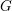$G$ be a reductive group over an algebraically closed subfield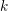$k$ of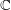$\mathbb{C}$ of characteristic zero,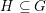$H\subseteq G$ an observable subgroup normalised by a maximal torus of$G$ and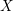$X$ an affine$k$ -variety acted on by$G$ . Popov and Pommerening conjectured in the late 1970s that the invariant algebra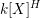$k[X]^{H}$ is finitely generated. We prove the conjecture for: (1) subgroups of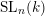$\operatorname{SL}_{n}(k)$ closed under left (or right) Borel action and for: (2) a class of Borel regular subgroups of classical groups. We give a partial affirmative answer to the conjecture for general regular subgroups of$\operatorname{SL}_{n}(k)$ .

## MSC classification

Type
Research Article
Information
Compositio Mathematica , January 2018 , pp. 36 - 79

## Access options

Get access to the full version of this content by using one of the access options below. (Log in options will check for institutional or personal access. Content may require purchase if you do not have access.)

## References

A’Campo-Neuen, A., Beitrage zur invariantentheorie reduktiver und unipotenter algebraischer Gruppen, PhD thesis, Basel (1994).Google Scholar
Bérczi, G., Doran, B., Hawes, T. and Kirwan, F., Geometric invariant theory for graded unipotent groups and applications, Preprint (2016), arXiv:1601.00340 [math.AG].Google Scholar
Bérczi, G., Doran, B., Hawes, T. and Kirwan, F., Projective completions of graded unipotent quotients, Preprint (2016), arXiv:1607.04181 [math.AG].Google Scholar
Bérczi, G., Doran, B., Hawes, T. and Kirwan, F., Constructing quotients of algebraic varieties by linear algebraic groups , in Handbook of group actions, Advanced Lectures in Mathematics (ALM), eds Ji, L., Papadopoulos, A. and Yau, S.-T. (Higher Education Press and International Press), to appear.Google Scholar
Borel, A., Linear algebraic groups, Graduate Texts in Mathematics, vol. 126 (Springer, New York, NY, 1991).CrossRefGoogle Scholar
Grosshans, F., Observable groups and Hilbert’s fourteenth problem , Amer. J. Math. 95 (1973), 229253.CrossRefGoogle Scholar
Grosshans, F., The invariants of unipotent radicals of parabolic subgroups , Invent. Math. 73 (1983), 19.CrossRefGoogle Scholar
Grosshans, F., Hilbert’s fourteenth problem for non-reductive groups , Math. Z. 193 (1986), 95103.CrossRefGoogle Scholar
Grosshans, F., Algebraic homogeneous spaces and invariant theory, Lecture Notes in Mathematics, vol. 1673 (Springer, New York, 1997).CrossRefGoogle Scholar
Grosshans, F., The invariant theory of unipotent groups, lecture notes (2010),http://www.matha.rwth-aachen.de/de/forschung/osalg/slides/UnipotentSlides.pdf.Google Scholar
Haboush, W., Reductive groups are geometrically reductive , Ann. of Math. (2) 102 (1975), 6784.CrossRefGoogle Scholar
Hochschield, G. and Mostow, G. D., Unipotent groups in invariant theory , Proc. Natl. Acad. Sci. USA 70 (1973), 646648.CrossRefGoogle Scholar
Leclerc, B. and Zelevinsky, A., Quasicommuting families of quantum Plücker coordinates , in Kirillov’s seminar on representation theory, American Mathematical Society Translations Series 2, Advances in Mathematical Sciences, vol. 181, ed. Olshanski, G. I. (American Mathematical Society, Providence, RI, 1998), 85108.Google Scholar
Magyar, P., Borel–Weil theorem for configuration varieties and Schur modules , Adv. Math. 134 (1998), 328366.CrossRefGoogle Scholar
Mumford, D., Fogarty, J. and Kirwan, F., Geometric invariant theory, Ergebnisse der Mathematik und ihrer Grenzgebiete, vol. 34, third edition (Springer, Berlin, New York, 1994).CrossRefGoogle Scholar
Nagata, M., Lectures on the fourteenth problem of Hilbert (Tata Institute, Bombay, 1965).Google Scholar
Pommerening, K., Invariant theory, Lecture Notes in Mathematics, vol. 1278, ed. Koh, S. (Springer, 1987), 817.CrossRefGoogle Scholar
Pommerening, K., Ordered sets with the standardizing property and straightening laws for algebras of invariants , Adv. Math. 63 (1987), 271290.CrossRefGoogle Scholar
Popov, V. L., Hilbert’s theorem on invariants , Dokl. Akad. Nauk SSSR 249 (1979), 551555.Google Scholar
Popov, V. L., Contraction of the actions of reductive algebraic groups , Math. USSR-Sb 58 (1987), 311.CrossRefGoogle Scholar
Seshadri, C. S., On a theorem of Weitzenbock in invariant theory , J. Math. Kyoto Univ. 1 (1961), 403409.CrossRefGoogle Scholar
Tan, L., On the Popov–Pommerening conjecture for the groups of type g 2 , Algebras Groups Geom. 5 (1988), 421432.Google Scholar
Tan, L., Some recent developments in the Popov–Pommerening conjecture , in Group actions and invariant theory, Conference Proceedings of the Canadian Mathematical Society, vol. 10 (American Mathematical Society, Providence, RI, 1989), 207220.Google Scholar
Tan, L., On the Popov–Pommerening conjecture for groups of type a n , Proc. Amer. Math. Soc. 106 (1989), 611616.Google Scholar
Weitzenbock, R., Über die invarianten von linearen gruppen , Acta Math. 58 (1932), 231293.CrossRefGoogle Scholar

# Send article to Kindle

Note you can select to send to either the @free.kindle.com or @kindle.com variations. ‘@free.kindle.com’ emails are free but can only be sent to your device when it is connected to wi-fi. ‘@kindle.com’ emails can be delivered even when you are not connected to wi-fi, but note that service fees apply.

Find out more about the Kindle Personal Document Service.

On the Popov–Pommerening conjecture for linear algebraic groups
Available formats
×

# Send article to Dropbox

To send this article to your Dropbox account, please select one or more formats and confirm that you agree to abide by our usage policies. If this is the first time you use this feature, you will be asked to authorise Cambridge Core to connect with your <service> account. Find out more about sending content to Dropbox.

On the Popov–Pommerening conjecture for linear algebraic groups
Available formats
×

# Send article to Google Drive

To send this article to your Google Drive account, please select one or more formats and confirm that you agree to abide by our usage policies. If this is the first time you use this feature, you will be asked to authorise Cambridge Core to connect with your <service> account. Find out more about sending content to Google Drive.

On the Popov–Pommerening conjecture for linear algebraic groups
Available formats
×
×